# Inferring chemical reaction patterns using rule composition in graph grammars

## Abstract

### Background

Modeling molecules as undirected graphs and chemical reactions as graph rewriting operations is a natural and convenient approach to modeling chemistry. Graph grammar rules are most naturally employed to model elementary reactions like merging, splitting, and isomerisation of molecules. It is often convenient, in particular in the analysis of larger systems, to summarize several subsequent reactions into a single composite chemical reaction.

### Results

We introduce a generic approach for composing graph grammar rules to define a chemically useful rule compositions. We iteratively apply these rule compositions to elementary transformations in order to automatically infer complex transformation patterns. As an application we automatically derive the overall reaction pattern of the Formose cycle, namely two carbonyl groups that can react with a bound glycolaldehyde to a second glycolaldehyde. Rule composition also can be used to study polymerization reactions as well as more complicated iterative reaction schemes. Terpenes and the polyketides, for instance, form two naturally occurring classes of compounds of utmost pharmaceutical interest that can be understood as “generalized polymers” consisting of five-carbon (isoprene) and two-carbon units, respectively.

### Conclusion

The framework of graph transformations provides a valuable set of tools to generate and investigate large networks of chemical networks. Within this formalism, rule composition is a canonical technique to obtain coarse-grained representations that reflect, in a natural way, “effective” reactions that are obtained by lumping together specific combinations of elementary reactions.

## Background

Directed hypergraphs  are a suitable topological representation of (bio)chemical reaction networks where (catalytic) reactions are hyperedges connecting substrate nodes to product nodes. Such networks require an underlying Artificial Chemistry  that describes how molecules and reactions are modeled. If molecules are treated as edge and vertex labeled graphs, where the vertex labels correspond to atom types and the edge labels denote bond types, then structural change of molecules during chemical reactions can be modeled as graph rewriting rules . In contrast to many other Artificial Chemistries this approach allows for respecting fundamental rules of chemical transformations like mass conservation, atomic types, and cyclic shifts of electron pairs in reactions. In general, a graph rewriting(rule) transforms a set of substrate graphs into a set of product graphs. Hence the graph rewriting formalism allows not only to delimit an entire chemical universe in an abstract but compact form but also provides a methodology for its explicit construction.

Most methods for the analysis of this network structure are directed towards this graph (or hypergraph) structure [1, 4], which is described by the stoichiometric matrix S of the chemical system. Since S is essentially the incidence matrix of the directed hypergraph, algebraic approaches such as Metabolic Flux Analysis and Flux Balance Analysis  have a natural interpretation in terms of the hypergraph. Indeed typical results are sets of possibly weighted reactions (i.e., hyperedges) such as elementary flux modes , extreme pathways , minimal metabolic behaviors  or a collection of reactions that maximize the production of a desired product in metabolic engineering. The net reaction of a given pathway is simply the linear combination of the participating hyperedges.

In the setting of generative models of chemistry, each concrete reaction is not only associated with its stoichiometry but also with the transformation rule operating on the molecules that are involved in a particular reaction. Importantly, these rules are formulated in terms of reaction mechanisms that readily generalize to large sets of structurally related molecules. It is thus of interest to derive not only the stoichiometric net reaction of a pathway but also the corresponding “effective transformation rule”. Instead of attempting to address this issue a posteriori, we focus here on the possibility of composing the elementary rules of chemical transformations to new effective rules that encapsulate entire pathways.

The motivation comes from the observation that string grammars are meaningfully characterized and understood by investigating the transformation rules. Consider, as a trivial example, the context-free grammar $G$ with the starting symbol S and the rules S → a Sa, S → a Sa | B and B → ε | b B, where ε denotes the empty string. Inspecting this grammar we see that we can summarize the effect of the productions as B → bk, k ≥ 0, and S → anB an, n ≥ 1. The language generated from $G$ is thus {anbkan|n ≥ 1, k ≥ 0}. Here we explore whether a similar reasoning, namely the systematic combination of transformation rules, can help to characterize the language of molecules that is generated by a particular graph rewriting chemistry. Similar to the example from term rewriting above, we should at the very least be able to recognize the regularities in polymerization reactions. We shall see below, however, that the rule based approach holds much higher promises.

Chemical reactions can be readily composed to “overall reactions” such as the net transformation of metabolic pathways. This observation is used implicitly in flux balance analysis at the level of the stoichiometric matrix. Recently,  considered the composition of concrete chemical reactions, i.e., transformations of complete molecules, as a means of reconstructing metabolic pathways. In this contribution we take a different point of view: instead of asking for concrete overall reactions, we are concerned with the composition of the underlying reaction mechanisms themselves. As we will see, these can be applied to arbitrary molecule contexts. We therefore address two issues: First we establish the formal conditions under which chemical transformation rules can be meaningfully composed. To this end, we discuss rule composition within the framework of concurrency theory in the following section. We then investigate the specific restrictions that apply to chemical systems, leading to a constructive approach for inferring composite rules.

The basic computational task we envision starts from an unordered set $R$ of reactions such as those forming a particular metabolic reaction pathway. To derive the effective transformation rule describing the pathway we need to find the correct ordering π in which the transformation rules p i , underlying the individual chemical reactions ρ i , have to be composed. We illustrate this approach in some detail using the Formose reaction as an example in the Results and discussion section.

## Graph grammars and rule composition

Graph grammars, or graph rewriting systems, are proper generalizations of term rewriting systems. A wide variety of formal frameworks have been explored, including several different algebraic ones rooted in category theory. We base our conceptual developments on the double pushout (DPO) formulation of graph transformations. For the comprehensive treatise of this framework we refer to . In the following sections we first outline the basic setup and then introduce full and partial rule composition. Alternative approaches to graph rewriting in the context of (artificial) chemistry have been based on the single pushout (SPO) model of graph transformations, see e.g. [11, 12]. We briefly discuss the rather technical difference between the DPO and SPO framework in Appendix 7, where we also briefly outline our reasons for choosing DPO.

### Double pushout and concurrency

The DPO formulation of graph transformations considers transformation rules of form $p=\left(L\stackrel{l}{←}K\stackrel{r}{\to }R\right)$ where L, R, and K are called the left graph, right graph, and context graph, respectively. The maps l and r are graph morphisms. The rule p transforms G to H, in symbols $G\stackrel{p,m}{⇒}H$ if there is a pushout graph D and a “matching morphism” m : L → G such that following diagram is valid: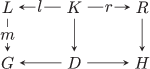The existence of D is equivalent to the so-called gluing condition, which determines whether the rule p is applicable to a match in G. In the following we will also write $G\stackrel{p}{⇒}H$ and GH for derivations, if the specific match or transformation rule is unimportant or clear from the context.

Concurrency theory provides a canonical framework for the composition of two graph transformations. Given two rules ${p}_{i}=\left({L}_{i}\stackrel{{l}_{i}}{←}{K}_{i}\stackrel{{r}_{i}}{\to }{R}_{i}\right)$, i = 1, 2, a composition $\left(L\stackrel{{q}_{l}}{←}K\stackrel{{q}_{r}}{\to }R\right)={p}_{1}{\ast }_{E}{p}_{2}$ can be defined whenever a dependency graph E exists so that in the following diagram: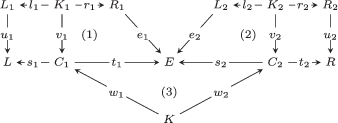the cycles (1) and (2) are pushouts, and (3) is a pullback, see e.g., . We then have q l  = s1w1 and q r  = t2w2. The concurrency theorem  ensures that for any sequence of consecutive direct transformations $G\stackrel{{p}_{1},{m}_{1}}{⇒}H\stackrel{{p}_{2},{m}_{2}}{⇒}{G}^{\prime }$ a graph E, a corresponding E-concurrent rule p1 E p2, and a morphism m can be found such that $G\stackrel{{p}_{1}{\ast }_{E}{p}_{2},m}{⇒}{G}^{\prime }$.

In order to use graph transformation as a model for chemical reactions additional conditions must be enforced. Most importantly, atoms are neither created, nor destroyed, nor transformed to other types. Thus only graph morphisms whose restriction to the vertex sets are bijective are valid in our context. In particular, the matching morphism m always corresponds to a subgraph isomorphism in our context. The context graph K thus is (isomorphic to) a subgraph of both L and R, describing the part of L that remains unchanged in R. Conservation of atoms means that the vertex sets of L, K, and R are linked by bijections known as the atom-mapping. When the atom mapping is clear, thus, we do not need to represent the context explicitly.

It is important to note that the existence of the matching morphism m : L → G alone is not sufficient to guarantee the applicability of the transformation. In our context, we require in addition that the transformation rule does not attempt to introduce an edge in R that has been present already before the transformation is applied. Formally, the gluing condition requires that (l(x), l(y)) L and (r(x), r(y)) R implies (m(l(x)), m(r(y))) G.

### Full rule composition

In the following we will be concerned only with special, chemically motivated, types of rule compositions. In the simplest case the dependency graph E is isomorphic to R1, later we will also consider a more general setting in which E is isomorphic to the disjoint union of R1 and some connected components of L2. For the ease of notation from now on we only refer to a rule composition, and not to a composition of morphisms as in the Graph Grammar section, i.e., p1 E p2 will be denoted as p2p1 (note the order of the arguments changes). If ER1, then L2e2(L2) is a subgraph of R1. Omitting the explicit references to the subgraph matching morphism e2 we can simply view L2 as subgraph of R1 as illustrated in Figure 1b.

The rule composition thus amounts to a rewriting ${R}_{1}\stackrel{{p}_{2},{e}_{2}}{⇒}R$, while the left side L1 is preserved. We will use the notation p2p1 and $G\stackrel{{p}_{2}\circ {p}_{1}}{⇒}H$ for this restricted type of rule composition, and call it full composition as the complete left side of p2 is a subgraph of R1. Note that L2 may fit into R1 in more than one way so that there may be more than one composite rule. Formally, the alternative compositions are distinguished by different matching morphisms e2 in the diagram in Figure 1a; we will return to this point below.

An example of a full rule composition is shown in Figure 1c. The two rules in the example, which in this case are also chemical reactions, are part of the Formose grammar. The Formose grammar consists of two pairs of rules. The first pair of rules, (from now on denoted as p0 and p1), implements both directions of the keto-enol tautomerism. One direction, p1, is visualized in Figure 1c. The second pair (from now on denoted as p2, p3) is the aldol-addition and its reverse respectively. The reverse (p3) is also visualized in Figure 1c. We see that the left side of p1 is isomorphic to a subgraph of one of the components of the right side of p3. Composing the two rules by subgraph matching yields a third rule, p1p3.

### Partial rule composition

An important issue for the application to chemical reactions is that the graphs involved in the rules are in general not connected. Typical chemical reactions combine molecules, split molecules or transfer groups of atoms from one molecule to another. The transformation rules for all these reactions therefore require multiple connected components. For the purpose of dealing with these rules, we introduce the following notation for graphs and derivations.

Let Q be a graph with # Q connected components Q i , $i=1,\dots ,\mathrm{#Q}$. It will be convenient to treat Q as the multiset of its components. A typical chemical graph derivation, corresponding to a bi-molecular reaction can be written in the form $\left\{{G}^{1},{G}^{2}\right\}\stackrel{p,m}{⇒}\left\{{H}^{1},{H}^{2},{H}^{3}\right\}$, where we take the notation to imply that all graphs Gi and Hj are connected.

The conditions for the composition of rules are a bit too strict for our applications. We thus relax them respect the component structure of left and right graphs. More precisely, we consider a partition of the components of L2 into two parts $\overline{{L}_{2}}$ and ${L}_{2}^{\prime }$ (cmp. Figure 2a), and we require that E is isomorphic to a disjoint union of a copy of R1 and ${L}_{2}^{\prime }$, while $\overline{{L}_{2}}$ must be isomorphic to a subgraph of R1. As a consequence, every connected component ${L}_{2}^{i}$ of L2 satisfies either ${e}_{2}\left({L}_{2}^{i}\right)\subseteq {e}_{1}\left({R}_{1}\right)$ or ${e}_{2}\left({L}_{2}^{i}\right)$ is a connected component of E isomorphic to ${L}_{2}^{i}$. For a rule composition of this type to be well defined we need that i such that ${e}_{2}\left({L}_{2}^{i}\right)\subseteq {e}_{1}\left({R}_{1}\right)$ holds, i.e., $\overline{{L}_{2}}$ must be non-empty. We remark that the latter condition could be relaxed further to lead to additional compositions for which left and right sides are disjoint unions. If ${L}_{2}^{\prime }$ is empty, then the partial composition is also a full composition.

As an abstract example (Figure 2a), the partial composition of p1 = (L1, K1, R1) and ${p}_{2}=\left(\left\{{L}_{2}^{2},{L}_{2}^{2}\right\},{K}_{2},{R}_{2}\right)$, with $\overline{{L}_{2}}={L}_{2}^{1}$ and ${L}_{2}^{\prime }={L}_{2}^{2}$ yields ${p}_{2}\circ {p}_{1}=\left(\left\{{L}_{1},{L}_{2}^{2}\right\},K,R\right)$. Note that right graph R cannot no longer be regarded simply as a rewritten version of R1 because rule p2 now adds additional vertices to both the left and the right graph. The composite context K contains only subsets of K1 and K2, but it is expanded by the vertices of ${L}_{2}^{2}$ and the edges of ${L}_{2}^{2}$ that remain unchanged under rule p2.

In general, we thus require here that the connected components of R1 and L2 satisfy either ${e}_{2}\left({L}_{2}^{i}\right)\subseteq {e}_{1}\left({R}_{1}^{\phantom{\rule{0.3em}{0ex}}j}\right)$ or ${e}_{1}\left({R}_{1}^{\phantom{\rule{0.3em}{0ex}}j}\right)\cap {e}_{2}\left({L}_{2}^{i}\right)=\varnothing$. We furthermore exclude the trivial case of parallel rules in which only the second alternative is realized. In other extreme, if all components ${L}_{2}^{i}$ satisfy ${e}_{1}\left({L}_{2}^{i}\right)\subseteq {e}_{2}\left({R}_{1}\right)$, the partial composition becomes a full composition. Formally, these alternatives are described by different dependency graphs E and/or different morphisms e1 and e2. Pragmatically we can understand this as a matching μ of L2 and R1 as in Figure 3. Specifying μ of course removes the ambiguity from the definition of the rule composition; hence we write p2 μ p1 to emphasize the matching μ.

## Constructing rule compositions

Given two rules, p1 and p2, it is not only interesting to know if a partial composition is defined, but also to create the set of all possible compositions

$\left\{{p}_{2}{\circ }_{{\mu }_{1}}{p}_{1},{p}_{2}{\circ }_{{\mu }_{2}}{p}_{1},\dots ,{p}_{2}{\circ }_{{\mu }_{k}}{p}_{1}\right\}$

explicitly. This set in particular contains also all full compositions. The following describes an algorithm for enumerating all partial compositions.

### Enumerating the matchings μ

The key to finding all compositions is the enumeration of all matchings μ that respect our restrictions on overlaps between connected components. We thus start from the sets $\left\{{R}_{1}^{1},{R}_{1}^{2},\dots ,{R}_{1}^{#{R}_{1}}\right\}$ and $\left\{{L}_{2}^{1},{L}_{2}^{2},\dots ,{L}_{2}^{#{L}_{2}}\right\}$ of connected components of R1 and L2, resp. In the first set we find all subgraph matches ${L}_{2}^{i}\subseteq {R}_{1}^{\phantom{\rule{0.3em}{0ex}}j}$ (represented as the corresponding matchings μ ij ) and arrange the result in a matrix of lists of subgraph matches, Figure 4.

The matching matrix is extended by a virtual column to account for the possibility that ${L}_{2}^{i}$ is not matched with any component of R1. Every partial (and full) composition is now defined by a selection of one submatch from each row of the matrix, see Appendix 7 for an example. The converse is not true, however: Not every selection of matches correspond to a partial composition. In particular, we exclude the case that only entries from the virtual column are selected. In addition, the submatches must be disjoint to ensure that the combined match is injective. The latter conditions needs to be checked only when more than one submatch is selected from the same column.

### Composing the rules

The construction of the composition p2 μ p1 of two rules p1 and p2 does not explicitly depend on the component structure of R2 and L1 because it is uniquely defined by the matching μ and the bijections of the nodes of L i , K i , and R i for each of the two rules. We obtain L by extending L1 with unmatched components of L2 and R by extending R2 by the unmatched components of R1. The corresponding extension of μ to a bijection $\stackrel{̂}{\mu }$ of the vertex sets of L and R is uniquely defined. The context K of the composite rule simply consists the common vertex set of L and R and all edges (x,y) of L for which $\left(\stackrel{̂}{\mu }\left(x\right),\stackrel{̂}{\mu }\left(y\right)\right)$ is an edge in R. We note in passing that $\stackrel{̂}{\mu }$ defines the atom mapping of the composite transformation. The explicit construction of (L, K, R) is summarized as the algorithm in Figure 5.

The implementation of the algorithm naturally depends heavily on the representation of transformation rules, which in our implementation is the representation from the Graph Grammar Library (GGL) . The representation is a single graph, with attached vertex and edge properties defining membership of L, K and R, as well as the needed labels.

Not all matchings define valid rule compositions. For instance, consider an edge (u, v) that is present in R1 and R2 but not in L2 and both u and v are in L2. This would amount to creating the edge by means of rule p2 which was already introduced by p1. Since we do not allow parallel edges and thus regard such inconsistencies as undefined cases we reject the matching. Note that a parallel edge does not correspond to a “double bond” (which essentially is only an edge with a specific type).

### Graph binding, unbinding, and identity

The composition of transformation rules, and thereby chemical reactions, makes it possible to create abstract meta-rules in a way that is similar to the combination of multiple functions into more abstract functions in functional programming. A related concept from (functional) programming that seems useful in the context of graph grammars is partial function application. Consider, for example, the binding of the number 2 to the exponentiation operator, yielding either the function f(x) = 2x or f(x) = x2. In the framework of rule composition, we define graph binding as a special case.

Let G be a graph and p2 = (L2, K2, R2) be a transformation rule. The binding of G to p2 results in the transformation rule p = (L, K, R) which implements the partial application of p2 on G. This is accomplished simply by regarding G as a rule p1 = (, , G), and using partial composition; p = p2p1. Note that if p2p1 is a full composition, then p can be regarded as a graph H and $G\stackrel{{p}_{2}}{⇒}H$ holds.

Graph binding allows a simplified representation of reactions. For instance, we can use this formal construction to omit uninteresting ubiquitously present molecules such as water by binding the graph of the water molecule to the transformation rule of a reaction that requires water. Similarly, graph unbinding can be defined as a transformation rule that destroys graphs. In a chemical application it can be used to avoid the explicit representation of uninteresting ubiquitous molecules such as the solvent.

As a direct consequence of graph binding and unbinding the composed rules create or destroy vertices. The atom map thus is not well-defined any more in such rules. This can be rescued by a formally different construction. We introduce the identity rule ı G  = (G, G, G). Instead of graph binding, we now consider the composed rules of form p2ı G  = (L, K, R) for some chemical rule p2. Its left graph must match G at least partially. This construction preserves a well-defined atom map and retains useful properties of graph binding. In particular, it ensures that G is a subgraph of the host graph in any derivations using p2ı G . Semantically, however, G is not bound but the constraint of its presence is. Since p2ı G admits an atom map and identifies G as a subgraph of L, there are two injective maps V(LG) → V(R) and V(G) → V(R) with disjoint images. The former identified the a partial atom map associated with the partial rule, that latter identified as atoms introduced by the bound molecules when p2ı G is applied to a substrate graph.

A technical issue worth mentioning is that partial rule composition as defined here can break the inherent symmetry of the DPO formalism in the sense that for p2p1 we cannot conclude that ${p}_{2}^{-1}$ satisfies the preconditions to be partially composed with ${p}_{1}^{-1}$ because their partial match of L2 with R1 does not necessarily respect component structure of R1 as required in our definition. The chemistry remains unaffected since reverse reactions are explicitly represented as different reactions.

### Ordering rules

A wide variety of methods, including flux balance analysis, can be used to identify pathways or other subsets of reactions that are of interest. Adjacency of reactions in the original networks as well as their directionality can be used efficiently to prune the possible orders of rule compositions. The fact that multiple reactions are instantiations of the same transformation rule, as in the example discussed in detail in the next section, further reduces the search spaces.

## Results and discussion

We illustrate the use of transformation rule composition by deriving meta-rules from the graph grammar consisting of the four rules necessary to represent the complete Formose reaction. The transformation rules for this system, p i , 0 ≤ i ≤ 3, are described in Figure 1 and in section “Full rule composition”. The influx and outflux molecules of the reaction is formaldehyde (g0) and glycolaldehyde (g1), and the reaction network for the complete reaction is depicted in 6.

Each reaction ρ i in the network is labeled with i and the instantiated transformation rule p j . That is, we have four well-known chemical transformation pattern; forward keto-enol tautomerism (p0: ρ2, ρ4, ρ6), backward keto-enol tautomerism (p1: ρ7, ρ9), forward aldol addition (p2: ρ3, ρ5), and backward aldol addition (p3: ρ8). Additionally label the influx of glycolaldehyde as a reaction, ρ1.

The overall reaction of the Formose cycle is 2g0 + g1 → 2g1, amounting to the linear combination $\sum _{i=2}^{9}{\rho }_{i}$ of the eight inner reactions of the network.

In this section we will not explicitly distinguish between partial composition and full composition, and we interpret the composition operator as right-associative to simplify the notation. Thus p i p j p k means p i  (p j p k ).

The rules are used in the autocatalytic cycle in the following order (starting with an keto-enol tautomerisation p0):

${p}_{0},{p}_{2},{p}_{0},{p}_{2},{p}_{0},{p}_{1},{p}_{3},{p}_{1}$

As it is not possible to compose this sequence of rules directly, we start with the identity rule for glycolaldehyde g1, as the before-mentioned keto-enol tautomerisation is applied to molecule g1. That is, we define the rule ${ı}_{{g}_{1}}=\left(\phantom{\rule{0.8pt}{0ex}}{g}_{1},{g}_{1},{g}_{1}\right)$ (see section “Graph binding, unbinding, and identity”). This rule, ${ı}_{{g}_{1}}$, is depicted in row 1 in the table in Figure 6.

Following the reaction sequence of the Formose cycle we subsequently compose with the transformation rules p i , 0 ≤ i ≤ 3, thus obtaining meta-rules modeling the prefixes of the overall reaction. These meta-rules are shown in row 2 to 9 in the table in Figure 6, with the final rule being a result of the composition ${p}_{1}\circ {p}_{3}\circ \dots \circ {p}_{0}\circ {ı}_{{g}_{1}}$. This rule precisely covers the reaction pattern of the Formose reaction, namely how two formaldehyde molecules and one glycolaldehyde are transformed to two glycolaldehyde molecules. However note, that the rule is general enough such that any pair of molecules with aldehyde groups can be used, i.e., the inferred reaction pattern refers to a class of overall reactions and the product does not necessarily need to be glycolaldehyde.

The composition starts with the identity rule ${ı}_{{g}_{1}}$, but a similar composition can be made by binding glycolaldehyde, i.e., using the rule (, , g1) as the starting rule. This results in rules which have the creation of atoms corresponding to glycolcaldehyde embedded. An example of such a composition is shown in Appendix 7.

The practical computation of these compositions takes less than a second in the current implementation. Even for substantially more general composition sequences the running time remains manageable. For instance, it takes less than 1 minute to compute all composition sequences with a length k ≤ 10 of the form ${\mathsf{p}}_{{i}_{1}}\circ {\mathsf{p}}_{{i}_{2}}\circ \cdots \circ {\mathsf{p}}_{{i}_{k}}\circ {ı}_{{g}_{q}}$ with i j  {0, 1, 2, 3}, based on the composition with the identity rule of one of the influx molecules, i.e., formaldehyde or glycolaldehyde. This results in 1875 different inferred composite rules.

Polymerization can also be viewed as a pathway in a chemical reaction network, albeit one of potentially infinite size. The same methods applied to the automatic inference of the overall reaction pattern of the Formose cycle can be directly applied to detecting composition rules for polymerization reactions. Importantly, even if a chemical reaction network is not given, the approaches presented in this paper can be used to automatically find sequences of reactions that will lead to polymerization. This can be realized by a straight-forward post-processing step: all that needs to be done is to check whether an inferred composite rule exhibits a replicated functional unit. Such polymerization meta-rules also enable the analysis of chemical systems with highly complex carbon skeletons such as the natural compound classes of the terpenes or the polyketides.

## Conclusions

Graph grammars provide a convenient framework for modeling chemistries on different levels of abstraction. A chemically valid approach is to see any chemical reaction as a bi-molecular reaction. This requires graph grammar rules that cover changes of molecules in an rather explicit and detailed way. Understanding chemical reaction patterns usually requires spanning the chemical reaction networks based on such rules. Obviously, this approach suffers the inherent potential of an immense combinatorial explosion. In this paper we introduced the automatic inference of such higher-level chemical reaction pattern based on a formal approach for graph grammar rule combination. We analyzed the autocatalytic cycle of the Formose reaction and inferred its overall reaction pattern as a rule composition of nine rules. Rule composition is also naturally applicable to inferring patterns of polymerization reactions. Future work will include e.g. the analysis of terpene-based and hydrogen cyanide-based polymerization chemistry. Many of the enzyme reactions collected in metabolism databases such as KEGG or MetaCyc are in fact overall reactions of multi-step mechanisms. The enzyme D-alanine transaminase (EC 2.6.1.21), for instance, achieves its chemical transformation in 12 elementary steps. Generating chemically correct atom-mappings of such overall reactions, a very important step e.g. in the interpretation of isotope tracer experiments, is infeasible with the currently available methods. In contrast a composite rule constructed from the individual enzymatic steps, as found for instance in the MACiE database , is guaranteed to yield the chemically correct atom mapping for the overall enzyme reaction.

## Appendix A: SPO and DPO in artificial chemistry models

In the double pushout (DPO) framework a production $L\stackrel{l}{←}K\stackrel{r}{\to }R$ is defined by three graphs (the left graph L, the right graph R, and the context graph K) and two graph morphisms l : K → L and r : K → R. In the single pushout (SPO) formalism, $L\stackrel{p}{↣}R$ is specified by only two graphs L and R and a partial graph morphism p. In other words, the production is specified by a total graph morphism $dom\left(p\right)\to R$, where $dom\left(p\right)$ is a subgraph of L. While DPO lives on the category of graphs and graph morphisms, SPO is built upon the category of graphs and partial graph morphisms, see  for a detailed comparison of the two approaches.

There is no real difference between the rules themselves since DPO and SPO productions can be translated into each other: $L\stackrel{p}{↣}R$ translates to $L\stackrel{l}{←}dom\left(p\right)\stackrel{r}{\to }R$ where l is the inclusion of $dom\left(p\right)$ in L and r is the domain restriction of p to $dom\left(p\right)$. Conversely, $L\stackrel{l}{←}K\stackrel{r}{\to }R$ translates to $L\stackrel{p}{↣}R$ where the partial morphism p = rl−1 is well-defined provided l is injective and $dom\left(p\right)=l\left(K\right)$.

The main difference between the two frameworks lies in the application of the productions, i.e., in the resulting (direct) graph derivations. SPO derivations are complete, that is, for each production $L\stackrel{p}{↣}R$ and each match $m:L\stackrel{m}{\to }G$ there is a derivation $G\stackrel{p,m}{⇒}H$. In contrast, in DPO the corresponding derivation exists if and only if the gluing condition is satisfied:

1. (I)

There are not distinct elements x,y of L with m(x) = m(y) and yl(K).

2. (D)

No edge e of Gm(L) is incident to a node in m(Ll(K)).

SPO is therefore more powerful than DPO in the sense that more general transformations can be implemented. On the other hand, the gluing condition ensures that there are no “side effects” such as dangling edges (which have to be eliminated by construction in SPO). These side effects make SPO transformation more difficult to understand and control in practical applications.

A useful feature of DPO derivations is that productions are invertible . As in chemical reactions, it suffices to exchange the roles of the left and the right graph, i.e., of products and educts. In contrast, the more general SPO derivations are not invertible in general.

In applications of graph transformation systems to modeling chemical reactions further restrictions are needed to account for the peculiarities of chemical transformation systems:

1. (i)

Reaction rules specify subgraphs. Therefore, the matching morphisms m and n are injective.

2. (ii)

Since atoms are conserved in chemical reactions, the restrictions l V and r V to the vertex set are bijections and determine the atom mapping. Edges model chemical bonds specified by electron pairs. These can only be moved around in the molecules but not collapsed onto the same bond with the same type. The morphisms l and r therefore must be injective.

### Lemma 1

Conditions (i) and (ii) imply the gluing condition.

### Proof

Since m is injective, i.e., m(x) = m(y) implies x = y, condition (I) is satisfied. Condition (ii) implies that l V (K) is the vertex set of L, i.e., Ll(K) contains no vertices. Thus m(Ll(K)) is empty so that condition (D) is trivially satisfied. □

As far as models of chemistry are concerned, therefore, SPO and DPO graph transformations are equivalent.

We prefer to work with the DPO framework for several reasons. First, the explicit exposure of the context graph K provides a convenient starting point for considering transition states e.g. in terms of the pair of subgraphs (Ll(K),Rr(K)). In addition, in our experience it is helpful to explicitly construct K in the process of designing rule sets of particular types of chemistry such as Diels Alder reactions or aldol condensations appearing in this contribution. Maybe more importantly, the DPO framework appears more convenient when building analysis tools such as coarse graining operations into the rewriting system. Finally, the framework of (injective) graph morphisms, in our view, is a more convenient basis for mathematical investigations than the partial graph morphisms on which SPO is built.

## Appendix B: Composition of the Formose reaction using graph binding

This Appendix illustrates the composition sequence from Figure 6 with a different starting rule. In Figure 6, the identity rule for glycolaldehyde ${ı}_{{g}_{1}}=\left({g}_{1},{g}_{1},{g}_{1}\right)$ is used, while here, Figure 7, we use the binding rule for the same molecule; (, , g1).

## Appendix C: Example of enumeration of compositions

In this Appendix we show the complete result of the composition of two (artificial) rules, p1 and p2, including the selection of submatches from the match matrix. The two rules are depicted in Figure 8 with the extended match matrix of the composition p2p1, that corresponds to the example of an extended match matrix as given in the paper. The rules in this section are all depicted with vertices that have an additional index. The numbering of the components is in increasing order wrt. to these indices, e.g., ${L}_{2}^{1}$ denotes the component connecting nodes A, 0 and B, 1 and ${L}_{2}^{2}$ denotes the component connecting nodes B, 2 and C, 3.

In the following we will enumerate all valid selections of submatches based on the extended match matrix and give the corresponding resulting rule composition. The chosen matches are depicted as ∙ in the extended match matrix. If several matches can be found (in our example this is true for the component ${L}_{2}^{1}$, that can be matched twice in ${R}_{1}^{3}$), the ∙ has an index.

### Composition 1Submatch selection in match matrix and result of composition 1.

### Composition 2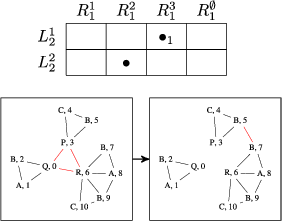Submatch selection in match matrix and result of composition 2.

### Composition 3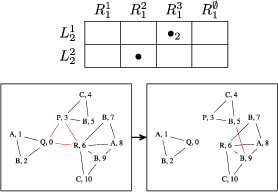Submatch selection in match matrix and result of composition 3.

### Composition 4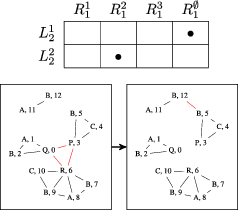Submatch selection in match matrix and result of composition 4.

### Composition 5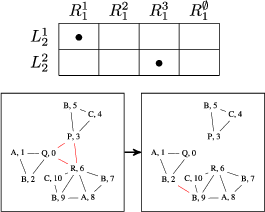Submatch selection in match matrix and result of composition 5.

### Composition 6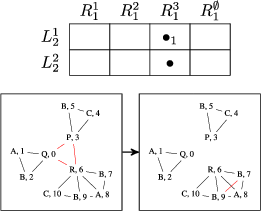Submatch selection in match matrix and result of composition 6.

### Invalid selectionThis selection of submatches is invalid, as they are not disjoint (node B, 9 would be matched twice).

### Composition 7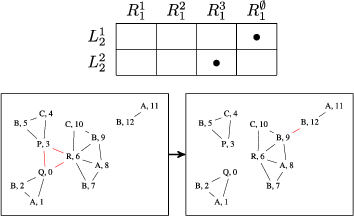Submatch selection in match matrix and result of composition 7.

### Composition 8Submatch selection in match matrix and result of composition 8.

### Composition 9Submatch selection in match matrix and result of composition 9.

### Composition 10Submatch selection in match matrix and result of composition 10.

## References

1. Klamt S, Haus UU, Theis F: Hypergraphs and cellular networks. PLoS Comput Biol 2009,5(5):e1000385. 10.1371/journal.pcbi.1000385

2. Dittrich P, Ziegler J, Banzhaf W: Artificial chemistries – a review. Artif Life 2001,7(3):225–275. 10.1162/106454601753238636

3. Benkö G, Flamm C, Stadler PF: A graph-based toy model of chemistry. J Chem Inf Comput Sci 2003,43(4):1085–1093. 10.1021/ci0200570

4. Aittokallio T, Schwikowski B: Graph-based methods for analysing networks in cell biology. Brief Bioinform 2006,7(3):243–255. 10.1093/bib/bbl022

5. Orth JD, Thiele I, Palsson BØ: What is flux balance analysis? Nature Biotech 2010, 28: 245–248. 10.1038/nbt.1614

6. Schuster S, Fell DA, Dandekar T: A general definition of metabolic pathways useful for systematic organization and analysis of complex metabolic networks. Nature Biotech 2000, 18: 326–332. 10.1038/73786

7. Price ND, Reed JL, Papin JA, Wiback SJ, Palsson BØ: Network-based analysis of metabolic regulation in the human red blood cell. J Theor Biol 2003, 225: 185–194. 10.1016/S0022-5193(03)00237-6

8. Larhlimi A, Bockmayr A: A new constraint-based description of the steady-state flux cone of metabolic networks. Discr Appl Math 2009, 157: 2257–2266. 10.1016/j.dam.2008.06.039

9. Félix L, Rosselló F, Valiente G: Efficient reconstruction of metabolic pathways by bidirectional chemical search. Bull Math Biol 2009, 71: 750–769. 10.1007/s11538-008-9380-8

10. Ehrig H, Ehrig K, Prange U, Taenthzer G: Fundamentals of Algebraic Graph Transformation. Berlin: Springer-Verlag; 2006.

11. Rosselló F, Valiente G: Graph transformation in molecular biology. Lect Notes Comp Sci 2005, 3393: 116–133. 10.1007/978-3-540-31847-7_7

12. Danos V, Feret J, Fontana W, Harmer R, Hayman J, Krivine J, Thompson-Walsh C, Winskel G: Graphs, rewriting and pathway reconstruction for rule-based models. Leibniz Int Proc Inf 2012. in press

13. Golas U: Analysis and Correctness of Algebraic Graph and Model Transformations. Wiesbaden: Vieweg+Teubner; 2010.

14. Ehrig H, Habel A, Kreowski HJ, Parisi-Presicce F: Parallelism and concurrency in high-level replacement systems. Math Struct Comp Sci 1991, 1: 361–404. 10.1017/S0960129500001353

15. Flamm C, Ullrich A, Ekker H, Mann M, Hogerl D, Rohrschneider M, Sauer S, Scheuermann G, Klemm K, Hofacker I, Stadler PF: Evolution of metabolic networks: a computational frame-work. J Syst Chem 2010,1(1):4. 10.1186/1759-2208-1-4

16. Mann M, Ekker H, Flamm C: The graph grammar library – a generic framework for chemical graph rewrite systems. 2013.http://arxiv.org/abs/1304.1356 []

17. Hompage of the Graph Grammar Library (GGL) [The source code and the documentation of the library can be accessed via, http://www.tbi.univie.ac.at/software/GGL/] [The source code and the documentation of the library can be accessed via, ]

18. Kanehisa M, Goto S, Sato Y, Furumichi M, Tanabe M: KEGG for integration and interpretation of large-scale molecular data sets. Nucleic Acids Res 2012, 40: D109–114. 10.1093/nar/gkr988

19. Caspi R, Altman T, Dreher K, Fulcher CA, Subhraveti P, Keseler IM, Kothari A, Krummenacker M, Latendresse M, Mueller LA, Ong Q, Paley S, Pujar A, Shearer AG, Travers M, Weerasinghe D, Zhang P, Karp PD: The MetaCyc database of metabolic pathways and enzymes and the BioCyc collection of pathway/genome databases. Nucleic Acids Res 2012, 40: D742–753. 10.1093/nar/gkr1014

20. Holliday GL, Andreini C, Fischer JD, Rahman SA, Almonacid DE, Williams ST, Pearson WR: MACiE: exploring the diversity of biochemical reactions. Nucleic Acids Res 2012, 40: D783–789. 10.1093/nar/gkr799

21. Ehrig H, Heckel R, Korff M, Löwe M, Ribeiro L, Wagner A, Corradini A: Algebraic approaches to graph transformation part II: single pushout approach and comparison with double pushout approach. In Handbook of Graph Grammars and Computing by Graph Transformations. Edited by: Rozenberg G. Singapore: World Scientific; 1997:247–312.

22. Löwe M: Algebraic approach to single-pushout graph transformation. Theor Comp Sci 1993, 109: 181–224. 10.1016/0304-3975(93)90068-5

23. Ehrig H: Introduction to the algebraic theory of graph grammars. Lect Notes Comp Sci 1979, 13: 1–69.

## Acknowledgements

This work was supported in part by the Volkswagen Stiftung proj. no. I/82719, the COST-Action CM0703 “Systems Chemistry”, and the Danish Council for Independent Research, Natural Sciences. Finally, we would like to thank the anonymous reviewers for their helpful comments and suggestions to improve the manuscript.

## Author information

Authors

### Corresponding authors

Correspondence to Christoph Flamm or Daniel Merkle.

### Competing interests

The authors declare that they have no competing interests.

### Authors’ contributions

JLA implemented the rule composition system. All authors contributed to the theory and the writing of the manuscript. All authors read and approved the final manuscript.

## Authors’ original submitted files for images

Below are the links to the authors’ original submitted files for images.

## Rights and permissions

Reprints and Permissions

Andersen, J.L., Flamm, C., Merkle, D. et al. Inferring chemical reaction patterns using rule composition in graph grammars. J Syst Chem 4, 4 (2013). https://doi.org/10.1186/1759-2208-4-4

• Accepted:

• Published:

• DOI: https://doi.org/10.1186/1759-2208-4-4

### Keywords

• Transformation Rule
• Graph Transformation
• Flux Balance Analysis
• Rule Composition
• Graph Grammar